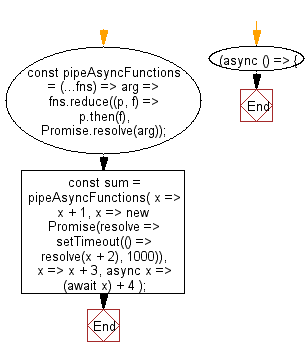# JavaScript: Perform left-to-right function composition for asynchronous functions

## JavaScript fundamental (ES6 Syntax): Exercise-166 with Solution

Write a JavaScript program to perform left-to-right function composition for asynchronous functions.

• Use Array.prototype.reduce() and the spread operator (...) to perform function composition using Promise.prototype.then().
• The functions can return a combination of normal values, Promises or be async, returning through await.
• All functions must accept a single argument.

Sample Solution:

JavaScript Code:

``````//#Source https://bit.ly/2neWfJ2
const pipeAsyncFunctions = (...fns) => arg => fns.reduce((p, f) => p.then(f), Promise.resolve(arg));
const sum = pipeAsyncFunctions(
x => x + 1,
x => new Promise(resolve => setTimeout(() => resolve(x + 2), 1000)),
x => x + 3,
async x => (await x) + 4
);
(async () => {
console.log(await sum(5)); // 15 (after one second)
})();
```
```

Sample Output:

```15
```

Flowchart:Live Demo:

See the Pen javascript-fundamental-exercise-166 by w3resource (@w3resource) on CodePen.

Improve this sample solution and post your code through Disqus

What is the difficulty level of this exercise?

Test your Programming skills with w3resource's quiz.

﻿